## Example Questions

### Example Question #6 : Complex Fractions

Simplify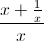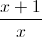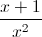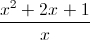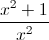Explanation:

Simplify the complex fraction by multiplying by the complex denominator: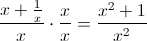### Example Question #23 : Problem Solving

Steven purchased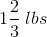of vegetables on Monday and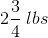of vegetables on Tuesday. What was the total weight, in pounds, of vegetables purchased by Steven?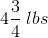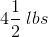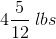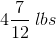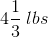Explanation:

To solve this answer, we have to first make the mixed numbers improper fractions so that we can then find a common denominator. To make a mixed number into an improper fraction, you multiply the denominator by the whole number and add the result to the numerator. So, for the presented data: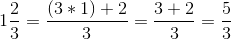and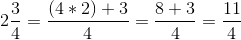Now, to find out how many total pounds of vegetables Steven purchased, we need to add these two improper fractions together: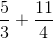To add these fractions, they need to have a common denominator. We can adjust each fraction to have a common denominator ofby multiplying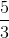by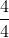and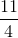by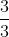: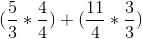To multiply fractions, just multiply across: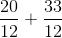We can now add the numerators together; the denominator will stay the same: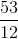Since all of the answer choices are mixed numbers, we now need to change our improper fraction answer into a mixed number answer. We can do this by dividing the numerator by the denominator and leaving the remainder as the numerator: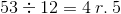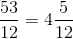This means that our final answer is.